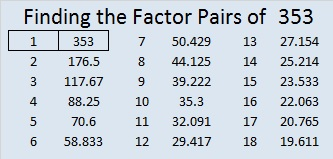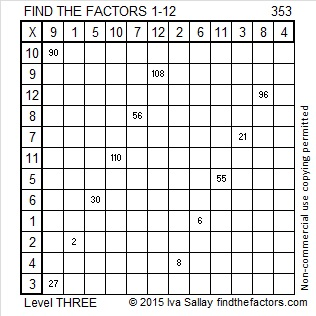# 353 and Level 3

When 2^353 is divided by 353, the remainder is 2, so 353 is VERY LIKELY a prime number. Scroll down past the puzzle to know for sure.Print the puzzles or type the factors on this excel file: 12 Factors 2015-01-12

• 353 is a prime number.
• Prime factorization: 353 is prime.
• The exponent of prime number 353 is 1. Adding 1 to that exponent we get (1 + 1) = 2. Therefore 353 has exactly 2 factors.
• Factors of 353: 1, 353
• Factor pairs: 353 = 1 x 353
• 353 has no square factors that allow its square root to be simplified. √353 ≈ 18.788How do we know that 353 is a prime number? If 353 were not a prime number, then it would be divisible by at least one prime number less than or equal to √353 ≈ 18.788. Since 353 cannot be divided evenly by 2, 3, 5, 7, 11, 13, or 17, we know that 353 is a prime number.

A Logical Approach to FIND THE FACTORS: Find the column or row with two clues and find their common factor. Write the corresponding factors in the factor column (1st column) and factor row (top row).  Because this is a level three puzzle, you have now written a factor at the top of the factor column. Continue to work from the top of the factor column to the bottom, finding factors and filling in the factor column and the factor row one cell at a time as you go.